STAT 330: 98-1

Assignment 1 Solutions

1. The most obvious source of probable bias is this: teachers are likely to move economically disadvantaged children to the group getting free milk. Typically these students will have the most to gain in educational or nutritional or behavioural ways. Thus the effect of the choice will be to increase the apparent effectiveness of a free milk program.
2. Let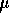be the average weight of a month's production of coffee. You are asked to choose between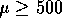and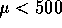the former being the manufacturer's claim. You have data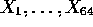, a sample from this population and observe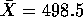. The manufacturer has also said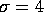so we will use this in our test.

To assess the evidence against the manufacturers claim you make that claim the null hypothesis: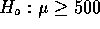and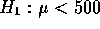. We use a z test (large sample size, known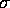) and get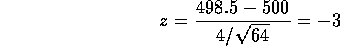The alternative predicts large negative z so the P-value is the area under the normal curve to the left of -3 or about 0.0013. The conclusion is that this P-value is so small that the manufacturer's claim is not credible; the packages are underweight.

3. Letbe the true concentration of cadmium in the lake. You are being asked to choose between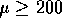and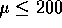. In this case you really ought to examine the data to see which, if either, of these possibilities is ruled out. Thus you can either see right off that the only possibility which might be ruled out isand make this the null or you can do both tests. Either way the t-statistic (small sample, hopefully normal population of concentration measurements) is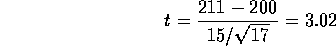For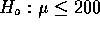you get P = 0.004 and for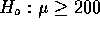you get P=.996. The first P-value means there is strong evidence againstwhile the second means there is little or no evidence against. The clear real world conclusion is that the concentration of cadmium in the lake is virtually certain to be over 200.

This conclusion summarizes the statistical calculations which rested on some assumptions. In practice there are serious potential problems which should be examined by the investigator (subject matter specialist not the statistician). Are the measurements unbiased? Are they independent or were they made in batches which would show more homogeneity within a batch than between? Are you really measuring the average concentration in the whole lake or only in a part of it?

4. Page 284, #4. Letbe the true average yield point of bars of the new composition. We are told that we have a sample of size 25 from a normally distributed population whose mean isand whose SD is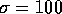.

1. The area to the left of 1.645 under a standard normal curve is 95% so a 90% confidence interval is given by the range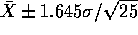or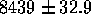.
2. The multiplier changes from 1.645 to 1.75.

5. Page 284, #6.

1. You must solve the inequalities as follows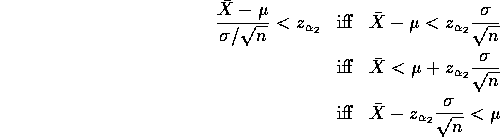and similarly for the other inequality to get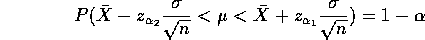so that the two things on the outside are the interval.

2. The lengths of the intervals are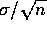times in one case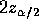and in the other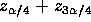. For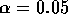these lengths are 3.92 and 4.02 so that the usual interval is shorter. (It is a theorem that using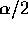produces the shortest interval.)

6. Page 290, #16. Let p be the true proportion of breast cancers which would be detected by this technique. Your model is that the number actually detected in the study, X, has a Binomial distribution with parameters p and n=29. It is not at all clear that the data really apply to any larger population of breast cancer patients - you have no idea how these 29 patients were chosen. You get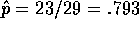and an approximate confidence interval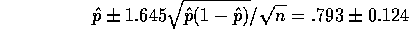which is, as the text warns, quite wide. The sample size is not terribly large and you might worry about the normal approximation but for a rough idea the normal approximation is ok.

7. Page 290, #18. Letbe the true average playing time of this population of tapes (in practice -- what population of tapes?). We havesampled from this population. We are given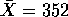and s=8 and asked to use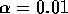from which the multiplier is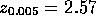or so. The confidence interval is then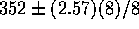or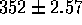. This interval does not contain 360 minutes so it is unlikely thatreally is 360. I think this data suggests strongly that the manufacturer is exaggerating. This question would be answered by a hypothesis whose z statistic is 8 leading to a miniscule P-value. There is no doubt whatever thatis less than 6 hours.
8. Page 314, #10.
1. The null hypothesis of correct calibration is that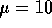and the alternative is that it is not.
2. The probability of recalibration is 1 minus the probability that the sample average is between 9.8968 and 10.1032, that is,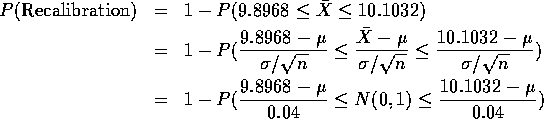In this part you are told to takeand get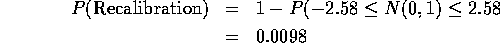3. Now you take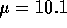and get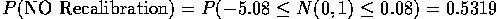and then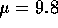and get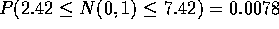.

4. You made this calculation in b above and got c=2.58.
5. Just by using n=10 in computing z. No change to c - that's the point of computing z.
6. This average can be computed by subtracting 10 and multiplying by 1000 to get the numbers -19, 6, -143, 107, -112, -207, -272, 439, 214 and 190 whose sum is 203 so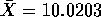with n=10 so that z = 0.32 and the hypothesis is not rejected at the 1% level.
7. Recalibrate if |Z| > 2.58.

Richard Lockhart
Tue Feb 10 12:18:18 PST 1998